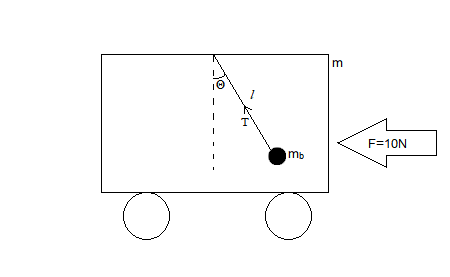# A cart of mass m (including the mass of the crane) has a pendulum hanging from a crane attached...

## Question:

A cart of mass m (including the mass of the crane) has a pendulum hanging from a crane attached to the cart. There is no friction due to the normal force, u = 0, or wheel bearings of the cart wheels. The cart is experiencing and applied force to the left of 10N. The ball at the end of massless rope attached to the crane has a mass mb and the length of the rope tied to the crane and ball is length, L. Express the motion of the ball in terms of m, mb,p (=10N), L and g (acceleration of gravity near the surface of the earth).

## The Inertial Frame of Reference:

In an example like a bob in hanging in a car, the frame of reference is assumed to be the car. When the car moves uniformly on a smooth horizontal surface, the car is said to be an inertial frame of reference. If the car changes its direction or the speed, the frame of reference(the car) accelerates. -This makes the car a non-inertial frame of reference. The objects within this frame of reference will experience a pseudo force that will act in the direction opposite the motion of the car.When the force is applied to the left, the bob will deviate towards the right. The angle by which the bob deviates depends on the acceleration experienced by the bob.

Applying Newton's Laws of Motion, we get

{eq}(m+m_b)a=F=10\\ a=\dfrac{10}{m+m_b} {/eq}

This acceleration will be experienced by the bob. The force balance equations for the bob will be

{eq}Tsin\theta=ma\\ Tcos\theta=mg {/eq}

Therefore, the relation between g and a will be

{eq}a=gtan\theta\\ or \ \ \ \dfrac{10}{m+m_b}=gtan\theta\\ \implies \theta=tan^{-1}\left(\dfrac{10}{(m+m_b)g}\right) {/eq}

The same equation can be rewritten as

{eq}x=l.tan^{-1}\left(\dfrac{10}{(m+m_b)g}\right) {/eq}

by multiplying {eq}l {/eq} on both sides. These are the equations of motion of the bob.